Previous Article in Journal
Thermal and Lighting Consumption Savings in Classrooms Retrofitted with Shading Devices in a Hot Climate
Open AccessArticle

# A Robust Control of Two-Stage Grid-Tied PV Systems Employing Integral Sliding Mode Theory

byAbbes Kihal,Fateh Krim *,Billel Talbi,Abdelbaset LaibandAbdeslem SahliLaboratory of Power Electronics and Industrial Control (LEPCI), Electronics Department, Faculty of Technology, University of Sétif1, Sétif 19000, Algeria
*
Author to whom correspondence should be addressed.
Energies 2018, 11(10), 2791; https://doi.org/10.3390/en11102791
Received: 26 August 2018 / Revised: 1 October 2018 / Accepted: 9 October 2018 / Published: 17 October 2018

## Abstract

This contribution considers an improved control scheme for three-phase two-stage grid-tied photovoltaic (PV) power systems based on integral sliding mode control (ISMC) theory. The proposed control scheme consists of maximum power point tracking (MPPT), DC-Link voltage regulation and grid current synchronization. A modified voltage-oriented maximum power point tracking (VO-MPPT) method based on ISMC theory is proposed for design of an enhanced MPPT under irradiation changes. Moreover, a novel DC-Link voltage controller based on ISMC theory is proposed to achieve good regulation of DC-Link voltage over its reference. To inject the generated PV power into the grid with high quality, a voltage-oriented control based on space vector modulation (SVM) and ISMC (VOC-ISMC-SVM) has been developed to control the grid current synchronization. Numerical simulations are performed in a MATLAB/SimulinkTM (R2009b, MathWorks, Natick, MA, USA) environment to evaluate the proposed control strategy. In comparison with conventional control schemes, the developed control strategy provides an accurate maximum power point (MPP) tracking with less power oscillation as well as a fast and an accurate DC-Link regulation under varying irradiation conditions. Moreover, the transfer of the extracted power into the grid is achieved with high quality.

## 1. Introduction

In recent years, photovoltaic (PV) power technology has become an important and promising source of energy due to its advantages such as cleanness, renewability, and non-noisiness [1,2]. To make PV power available for public use, two main topologies are used to transfer the produced PV power into the grid, called single and two stages . The latter topology has been extensively employed since maximum power point tracking (MPPT) and control of generated power transfer into the grid are decoupled by different converters. This feature offers an easier and better control than the single stage topology control [4,5]. To enhance the cost-effectiveness of this topology, maximum power point (MPP) should be tracked quickly and accurately under irradiation changes. Moreover, the DC-Link voltage and power injected into the grid must also be controlled accurately.
Many MPPT techniques have been proposed in the literature to push PV arrays to deliver the MPP under climatic condition changes [6,7,8,9,10,11,12,13,14,15,16,17,18,19,20,21,22,23]. Perturb and Observe (P&O) [7,8] and Incremental Conductance (INC) [9,10,11] are two well-known conventional MPPT techniques. They are intuitive to understand and easy to implement. However, they are not able to operate properly in case of fast changes in environmental conditions; afterwards, they present large oscillations at the MPP in steady-state conditions. In this context, artificial intelligence is adopted to overcome these drawbacks in the form of different methods such as Artificial Neural Networks (ANN) , Fuzzy Logic (FL) [13,14,15], Genetic Algorithms (GA) , Pattern Swarm Optimization (PSO) , and Grey Wolf Optimization (GWO) . Unlike conventional methods, the modern algorithms provide better performance. However, they are complex in design and rigorous to implement in practice. Therefore, several research works were interested in Voltage-Oriented MPPT (VO-MPPT) and Current-Oriented MPPT (CO-MPPT) [19,20,21,22,23,24]. These methods involve MPPT voltage-based algorithm (V-MPPT) in cascade with voltage control loop or MPPT current-based algorithm (C-MPPT) in cascade with current control loop. Due to the almost-zero change in PV voltage at the MPP under irradiation changes, VO-MPPT is considered to be a fast MPP tracking strategy [19,20]. However, the efficiency of this method depends on the voltage regulator design.
To ensure transfer of produced PV power into the grid, the DC-Link voltage must be regulated to a desired value higher than the grid peak voltage. The purpose of this regulation is to maintain the DC-Link voltage to its desired value as well as to estimate the reference power or current for the grid-side inverter controller. For this purpose, a proportional−integral (PI) or fuzzy controllers are widely used in the literature [24,25,26,27]. However, PI regulator suffers from long response time and inaccurate regulation under solar irradiation changes. To overcome the inherent difficulties of the PI controller, several types of fuzzy controllers have been proposed [24,25]. These intelligent controllers provide high-performance control. However, they lead to high computational burden which drastically reduces their use, especially in the case of complex systems.
Obviously, the injection of the produced PV power into the grid must be performed with low total harmonic distortion (THD %) for grid currents. In this context, numerous research works are interested in direct power control (DPC)  and virtual flux direct power control (VF-DPC)  based on switching tables. These two techniques do not provide high-performance control, and the switching frequency is variable and not controllable. Otherwise, some researchers have developed distinct control strategies such as hysteresis control  or Finite-set predictive control . Hence, these strategies provide high-performance control, but they suffer from uncontrollable switching frequency. Other research works have employed voltage-oriented control (VOC) based on PI controllers through pulse width modulation (PWM) or space vector modulation (SVM) . In these strategies, the PI controller weaknesses reduce the power transfer control performance.
Currently, sliding mode control (SMC) theory is widely introduced in power electronic converters control [33,34,35,36]. In grid-tied PV systems, SMC is employed for different purposes such as: to modify the voltage control loop of VO-MPPT [22,23], or internal current loop of VOC . The application of SMC offers high control performance in addition to simple experimental implementation [33,34,35,36,37]. However, it suffers from an undesirable effect named chattering. This problem is inevitably caused by the conventional switching function (signum function) which leads to high switching frequency. To reduce this phenomenon, numerous research works use the saturation as switching function . In [23,36] the error between the selected variable and its reference has been used as input for switching surface. However, a steady-state error (SSE) remains. That is why an advanced theory in SMC was developed to enhance the SMC control performance and reduce its disadvantage by adding an integral term to the sliding surface, which is called an integral sliding mode controller (ISMC).
In this paper, ISMC theory is used to develop effective and simple controllers for a two-stage grid-tied PV system. Moreover, the control law design has been modified, where an approximation of sign function is used as switching function resulting in a significant reduction of the chattering phenomenon. Firstly, a fixed switching frequency VO-MPPT based on ISMC theory is proposed to control the first stage (DC-DC converter) to achieve a fast and accurate MPP tracking under solar irradiation changes. Then, a new design of DC-Link voltage control based on ISMC theory is proposed to improve the regulation performance compared to other controller types, under linear solar irradiation changes. Furthermore, to control the injection of produced PV power into the grid with high grid current quality, a modified VOC based on ISMC theory is proposed. Numerical simulations through MATLAB/SimulinkTM and Simpower packages are carried out to confirm the improvement in control performance owing to the proposed scheme based on ISMC theory, under solar irradiation changes, in comparison with a conventional control scheme based on PI regulators.
Bearing these ideas in mind, the remainder of this paper is arranged as follows. The second section introduces an overview of the global system configuration. The proposed control scheme with detailed procedure design is presented in the third section. Simulation results and discussions are presented in the fourth section, after which conclusions are drawn in the final section.

## 2. Overall System Configuration

The system under consideration, which is shown in Figure 1, represents two-stage topology of the grid-tied PV system. It is mainly composed of the following blocks: PV array which converts solar irradiation into electrical energy, DC-DC boost converter is used to track the MPP and deliver it continuously to the DC-Link, two-level three-phase inverter whose role is to transfer the generated PV power from the boost converter to the mains grid through a passive filter .

#### 2.1. PV Array Model

The PV cell is the basic component in the conversion of sunlight into direct electrical energy. Several PV cells connected either in series or parallel form a PV array, which could be modeled by the following equation 
where, $i p h$ is the photocurrent, $i o$ the reverse saturation or leakage current of the diode, $R s P V$ and respectively the series and shunt resistances of the PV cell, the thermal voltage of the PV module, the diode ideality factor, $N s$ and $N P$ the numbers of PV cells in series and parallel respectively .
The PV array model is simulated for different irradiation values at T = 25 °C and for different temperature values with a fixed irradiation value G = 1000 W/m2, to show the effect of irradiation and temperature variations on the PV array. According to obtained (I–V) and (P–V) characteristics presented in Figure 2, a strong dependence links the PV array current to the irradiation which affects strongly the MPP of the PV array. Nevertheless, the voltage increases a little bit when the solar irradiation G is increased from 500 W/m2 to 1000 W/m2. In contrast, as shown in Figure 3, the temperature change has little effect on the MPP of the PV array. Also, it is worth noting that the temperature variations during the day are generally not important.

#### 2.2. Boost Converter Modeling

The DC-DC boost converter is usually used in PV systems for tracking the MPP produced by the PV array due to some of its important features, such as simplicity, robustness, and continuous input current. Figure 4 shows the equivalent boost circuits for both on and off switching states. The dynamic model of the boost converter in term of duty cycle $“ D ”$ can be expressed, using averaging method presented in , as
where, $i L$ and $v p v$ are the state variables; $D$ duty cycle considered as input variable; $v d c$ the DC-Link capacitor voltage.

#### 2.3. Inverter Model

The power circuit of the three-phase inverter consists of six bidirectional switches to connect the three-phase grid through a passive filter $“ R g , L g ”$. Each bidirectional switch is composed of an Insulated Gate Bipolar Transistor (IGBT) with antiparallel diode. The two switches of each inverter leg must operate in a complementary mode to avoid the short circuit of the DC-Link. The inverter voltage vector can be expressed in terms of DC-Link voltage and switching states as follows 
where $S a$, and are the switching states of the inverter.

## 3. Proposed Control Scheme

The proposed controllers for the two-stage grid-tied PV system are as follows:
• VO-MPPT based on ISMC, to enhance the PV energy conversion efficiency under any irradiation changes.
• DC-Link voltage controller based on ISMC, to maintain the DC-Link voltage close to its reference under any irradiation changes.
• VOC based on the modified ISMC and SVM, to control the injection of the produced PV power into the grid.

#### 3.1. ISMC Theory

The concept of an integral sliding mode controller (ISMC) generally includes three steps. The first step concerns the design of a sliding surface on which the sliding motion will take place. The control law is designed in the second step. The control law concept depends on the selection of switching function to force the system state trajectories to track and slide on the sliding surface. Whereas, the last step ensures the reaching condition, which pledges the existence of the sliding mode [38,39].
In the present contribution, a high-performance control scheme for grid-tied PV system using ISM controllers is proposed. In ISMC theory, an integral term is added to the sliding surface to obtain a high-performance control and to achieve a quick and accurate tracking. The sliding surface $“ s ”$ in an ISM controller can be expressed as
where, $e$ is the error between the measured variable and its reference, $k i$ is the sliding surface coefficient.
Typically, the control law has a structure given as
where, $u e q$ is the equivalent part responsible for helping to keep sliding, and $u d i s$ is the discontinuous part used to enforce the sliding mode to remain along the sliding surface traditionally written as follows
where, $M$ is the proportional gain of discontinuous control and is sign function.
The discontinuity related with this part leads to chattering phenomenon. To eliminate this drawback, the sign function is replaced with its smoothing approximation, as shown in Figure 5. Hence can be expressed by the following equation
where, $α$ is a small positive value.
After the design of control law in the previous steps, the conditions required to ensure the control stability will be checked. Lyapunov function is used to examine the control stability. It is defined as follows
Therefore, to guarantee the convergence of $V$, it should be verified that the derivative of $V$ is negative as follows
Accordingly, one will define individually the reaching condition for each of the three proposed controllers, in the following sections.

#### 3.2. MPPT Control

To obtain an optimal exploitation of the PV array during all solar irradiation changes, the proposed control scheme is divided in two steps as shown in Figure 6. Firstly, a P&O-based voltage MPPT generates the reference voltage $V r e f$. After that, an improved ISMC-based cascade voltage regulator is proposed to enforce the PV voltage $v p v$ to track $V r e f$ generated previously by providing the suitable duty cycle $D$ for PWM modulation stage to control the boost converter.

#### 3.2.1. P&O-Based V-MPPT

The objective of the V-MPPT is to generate $V r e f$ corresponding to the MPP. For this purpose, P&O-based V-MPPT technique is employed due to its featuring effectiveness and simplicity as shown in Figure 7.

#### 3.2.2. ISM Controller Design

Due to the almost-zero change in PV voltage at the MPP under irradiation changes, VO-MPPT is considered to be a fast alternative [18,19]. However, the efficiency of this method depends on the cascade voltage regulator concept. In this section, an enhanced cascade voltage regulator based on ISMC theory is proposed to provide the suitable duty ratio $D$ to drive the boost converter towards to improve the extracted power from the PV array. Hence, in the controller strategy the sliding surface has the same form as given in Equation (4) and expressed as
where, $e p v ( t )$ is the voltage error between $“ V r e f ”$ and $“ v p v ”$ and $k i , P V$ is the sliding surface coefficient.
It is considered that $δ$ is the sliding surface derivative, which is also called the sliding manifold, given as
To obtain the equivalent control signal, Equation (11) must be derived and equated to zero as follows
$δ ˙ = e ¨ p v + k i , P V e ˙ p v = 0 Or − v ¨ p v − k i , P V v ˙ p v = 0$
By using Equation (2), Equation (12) can be written as
Thus, the equivalent control can be expressed as
Hence, one part of the control input can be obtained; the second part called $D d i s$ is given by Equation (7).
Therefore, the overall sliding mode controller is expressed as
where, $M P V$ is the proportional gain of discontinuous control parts.
The controller described by Equation (15) must satisfy the reaching condition. From Equation (2), we obtain
By substituting $v ˙ p v$ and $v ¨ p v$ in Equation (12), we get
By substituting the overall control law $D$ given by Equation (15) in Equation (17) and after some simplifications, we get
Finally, the reaching condition must be verified, which means $δ · δ ˙ < 0$. Thus
Since $s p v ‖ s p v ‖ + α$ and $v d c L · C i n$ are always positive, it is obvious that the reaching condition is verified only if $M P V$ is chosen positive.

#### 3.3. Design of DC-Link Voltage Controller

As mentioned previously, the objective of DC-Link regulation is to maintain the DC-Link voltage close to its reference value and estimate the reference d-axis grid current $“ i d g * ”$ whatever the produced PV power. For this purpose, a novel design based on ISMC theory for the DC-Link voltage control is discussed in this section. The block diagram of the proposed DC-Link controller is shown in Figure 8.
The proposed sliding surface of the DC-Link voltage control is given below
where, $e d c$ is the voltage error between the DC-Link voltage $v d c$ and its reference value $V d c *$ and $k i , D C$ is the sliding surface coefficient.
The time derivative of the previous equation is given by
By considering energy conservation, it yields
where, , $p d c$ and $p g$ are the produced PV power, DC-Link power and power injected into the grid, respectively.
The injected power into the three-phase grid system (in synchronous d-q frame) is expressed as
The aim of the proposed controller is to inject only the produced PV power. According to this condition, the q-axis grid current becomes null and Equation (23) can be rewritten as
The inverter input power is given as
By applying Kirchhoff current law at the DC-Link between the inverter and boost converter it yields
where, is the boost output current, $i i n v$ inverter input current and $C d c$ is the DC-Link capacitor.
By substituting Equations (24) and (25) in Equation (22), it yields
By assuming that the DC-Link voltage $v d c$ is properly regulated, the derivative of the DC-Link voltage $v ˙ d c$ (or capacitor current $i d c$) becomes close to zero. Then Equation (26) can be written as
Then,
The current $i o u t$ can be determined as
By replacing Equations (27) and (30) in Equation (26), we get
To determine the equivalent control, Equation (21) must be set to zero as follows
From Equation (32) the equivalent control law is expressed as
Therefore, the global control law can be written as
where, $M D C$ is the proportional gain of discontinuous control part.
To confirm that the controller described by Equation (34) satisfies the reaching condition, $s ˙ d c$ needs to be evaluated.
By substituting Equation (31) in Equation (21), we get
By substituting the overall control law $i d g *$ given by Equation (34) in Equation (35), we get
As previously, the condition must be verified. Thus
Since $( s d c 2 ‖ s d c ‖ + α )$ and $( 3 v d g 2 C d c v d c )$ are positive, so it is clear that the reaching condition is verified only if $M D C$ is chosen positive.

#### 3.4. VOC Based ISMC

In this section, a modified VOC based on ISM control theory (VOC-ISMC) is presented and detailed. The main objective of the proposed control scheme is to inject the generated PV power into the grid with high grid current quality. VOC-ISMC is based on the calculation of the reference voltage vector which is applied through SVM in order to minimize the error between the rotating frame currents $i d g$, $i q g$ and their references $i d g *$,. Figure 9 summarizes the functionality of VOC-ISMC. Firstly, $i d g *$ is estimated by the DC-Link voltage regulator presented in the above section, while $i q g *$ is set to zero to eliminate the injection of reactive power into the grid. Then, the obtained reference voltage vector $( v d *$, $v q * )$ is transformed to α-β frame $( v α *$, $v β * )$ and applied through SVM to control grid-tied inverter. The phase-locked loop (PLL) allows controlling an estimated angle $θ g$ with respect of the grid phase angle and it is also needed for the Park’s transformation.
The development of the proposed control scheme needs the mathematical model of the grid-tied inverter in d-q rotating frame which can be described in terms of inverter voltages, grid voltages, and filter inductance  as
where $( v d g$, $v q g ) ,$ $( i d g$, , $V q )$ are the (d-q) components of grid voltages, grid currents and inverter output voltages respectively; $ω$ is the grid angular frequency; $R g$ and $L g$ are the resistance and inductance of the filter, respectively.
The proposed sliding mode surfaces are defined as follows
where, is the error between and $i d g$ currents, while $e q$ is the error between $i q g *$ and $i q g$ currents $k i , d$, $k i , q$ are constant gains.
The time derivative of the above equation yields
The equivalent control terms are obtained by setting $s ˙ d$ and to zero. Thus
By substituting Equation (38) in Equation (41), we get
The equivalent control terms are given by
The global control law is summarized as follows
where, $M d$ and $M q$ are proportional gains of the discontinuous control parts.
To confirm that the controller described in Equation (44) satisfies the reaching condition, the expressions of $s ˙ d$ and $s ˙ q$ are needed.
So, by substituting Equation (38) in Equation (40), we get
Then, by replacing the global control law ( and $v q *$) given by Equation (44) in Equation (45), we obtain
The reaching condition can be expressed as
By substituting Equation (46) in Equation (47), we obtain
As known that the terms $s d 2 ‖ s d ‖ + α$, $s q 2 ‖ s q ‖ + α$ and $1 L g$ are positive, the gains $M d$ and $M q$ must be chosen negative in order to satisfy the reaching condition of the controller described in Equation (48).

## 4. Simulation Results and Discussion

Numerical simulations using MATLAB/SimulinkTM environment are carried out to confirm the performance of the proposed scheme compared to the conventional scheme. The specifications are listed in the Appendix A. The DC-Link reference voltage is fixed at 220 V. The reference current $i q g *$ is set to zero (injecting only the active power). As discussed in Section 2.1, the temperature has a little effect on the MPP position of the PV array; in contrast, the irradiation level significantly affects the PV array performance. That is why the proposed control scheme is verified under suddenly and linearly varying irradiation as shown in Figure 10 by considering a constant temperature value of 25 °C. The proposed control scheme is compared with the conventional scheme (PI-based controller), with the same specifications in Appendix A to provide a fair comparison.
Figure 11 and Figure 12 represent the results obtained with the conventional and proposed control schemes, respectively. From top to bottom, the waveforms given in Figure 11 and Figure 12 are: (a) PV voltage, (b) PV current, (c) PV power, (d) DC-Link voltage with its desired reference, (e) $i d g$ current with its reference, (f) $i q g$ current with its reference.
At the outset, the results confirm the low tracking speed of the conventional control scheme (PI-based controller) under irradiation changes with significant PV voltage fluctuation in steady-state. In contrast, a high tracking performance was exhibited by using the proposed control scheme. Also, an instantaneous effect on the PV voltage is displayed under irradiation changes without overshoots and with less fluctuation, as shown in Figure 13. Due to the relationship between the PV power and PV voltage, the performance of MPP tracking is affected by the PV voltage behavior, when the PV voltage fluctuation and tracking time are small, the extracted PV power loss is minimized. Moreover, Figure 14 illustrates the improvement in the extracted PV power using the proposed control scheme compared to the conventional VO-MPPT. For more details, the main simulation results obtained from both MPPT methods are summarized in Table 1.
Furthermore, we examine the DC-Link voltage regulation using the conventional PI controller and the proposed ISMC scheme. The obtained results shown in Figure 15 confirm the tracking superiority of the proposed control scheme compared to the conventional one under solar irradiation changes. It can be observed, that by using the conventional PI controller, the DC-Link voltage deviates from its reference in case of linear changes in solar irradiation. Moreover, in case of step irradiation changes, the overshoot and response time are significantly large. In contrast, the desired reference is accurately tracked by employing the proposed ISMC in addition to, insignificant overshoot and short response time under step irradiation changes. To show the performance improvement by using the proposed control scheme compared to the conventional scheme, key results are presented in Table 2.
We also observe that the proposed VOC-ISMC scheme allows to inject the extracted PV power into the grid with high quality and efficiency, contrary to the conventional control scheme (VOC-PI controller). It can be observed that by applying the proposed control scheme, $i d g$ and $i q g$ currents are completely regulated to their references. It is found that $i d g$ current exhibits fast response with less fluctuation compared to the conventional control, as shown in Figure 12e, which leads to increase the quality of grid currents and their amplitudes almost instantaneously follow the irradiation changes, as shown in Figure 16b. In contrast, the grid current waveforms obtained by using the conventional control scheme are relatively of poor quality due to the large fluctuation and response time of $i d g$ current, as shown in Figure 16a. A comparison summary in terms of key indexes such as grid current THD% and $i d g$ current ripples for various irradiation levels is depicted in Figure 17.

## 5. Conclusions

This paper proposed a high-performance control scheme for a two-stage grid-tied PV system. The control structure of the proposed scheme is based on ISMC theory and consists of MPPT control, DC-Link voltage regulation loop, and VOC based on inner current control loops to improve system performance during all climatic conditions. As it is a two-stage system, the PV power is provided to the grid through a DC-DC boost converter and three-phase voltage source inverter. An ISM controller for the boost converter has been proposed for MPP tracking. Moreover, for proper inverter operation as well sinusoidal currents injection into the mains grid with low THD% (<5%), a novel design for DC-Link voltage regulation is suggested to maintain the DC-Link voltage constant at the desired value and estimate the reference currents for the VOC scheme, which is based on ISM controllers and space vector modulators. The simulation results obtained confirm the feasibility, effectiveness, and performance improvement of the proposed control scheme. As a future perspective, an implementation of the proposed control scheme using a field-programmable gate array (FPGA) control board will be introduced, where FPGA devices are generally cheaper and are suited for high-speed demanding application.

## Author Contributions

A.K. and B.T. designed and achieved the method; F.K. verified and analyzed the results; A.L. and A.S. wrote the paper.

## Funding

This research received no external funding.

## Conflicts of Interest

The authors declare no conflict of interest.

## Appendix A

The global specifications of the grid-tied PV system used in the simulation are listed in Table A1.
Table A1. Global system specifications.
Table A1. Global system specifications.
 GPV Nominal Values Open circuit voltage $( V O C )$ 42.1 [V] Optimum operating voltage $( V m p p )$ 33.7 [V] Short circuit current $( I S C )$ 3.87 [A] Optimum operating current $( I m p p )$ 3.56 [A] Maximum power $( P m p p )$ 120 [W] Number of cells connected in series $N s$ 72 Number of cells connected in parallel $N p$ 1 Number of panels connected in series $N s s$ 2 Number of panels connected in parallel 2 Boost converter Values Inductance $( L )$ 1 [mH] Input capacitor $( C i n )$ 470 [µF] output capacitor $( C d c )$ 200 [µF] Electrical parameters of grid-tied Values Output filter inductance $( L g )$ 10 [mH] Line resistance $( R g )$ 0.1 [Ω] Nominal grid frequency $( f g )$ 50 [Hz] Nominal line voltage of the 3-phase grid $( v g )$ 100 [V] Simulation parameters Values V-MPPT sampling frequency $( T s )$ 1 [kHz] PWM switching frequency $( f s , P W M )$ 5 [kHz] SVM switching frequency $( f s , S V M )$ 25 [kHz]

## References

1. Dincer, F. The analysis on photovoltaic electricity generation status, potential and policies of the leading countries in solar energy. Renew. Sustain. Energy Rev. 2011, 15, 713–720. [Google Scholar] [CrossRef]
2. Shayestehfard, A.; Mekhilef, S.; Mokhlis, H. IZDPWM based feed forward controller for grid connected inverters under unbalanced and distorted conditions. IEEE Trans. Ind. Electron. 2017, 64, 14–21. [Google Scholar] [CrossRef]
3. Xiao, W.; Edwin, F.F.; Spagnuolo, G.; Jatskevich, J. Efficient approaches for modeling and simulating photovoltaic power systems. IEEE J. Photovolt. 2013, 3, 500–508. [Google Scholar] [CrossRef]
4. Kanaan, H.; Caron, M.; Al-Haddad, K. Design and implementation of a two-stage grid-connected high efficiency power load emulator. IEEE Trans. Power Electron. 2014, 29, 3997–4006. [Google Scholar] [CrossRef]
5. Debnath, D.; Chatterjee, K. Two-stage solar photovoltaic-based stand-alone scheme having battery as energy storage element for rural deployment. IEEE Trans. Ind. Electron. 2015, 62, 4148–4157. [Google Scholar] [CrossRef]
6. Femia, N.; Petrone, G.; Spagnuolo, G.; Vitelli, M. Power Electronics and Control Techniques for Maximum Energy Harvesting in Photovoltaic Systems; CRC Press: Boca Raton, FL, USA, 2012. [Google Scholar]
7. Ahmed, J.; Salam, Z. An improved perturb and observe (P&O) maximum power point tracktwotwing (MPPT) algorithm for higher efficiency. Appl. Energy 2015, 150, 97–108. [Google Scholar]
8. De Brito, M.A.G.; Galotto, L.; Sampaio, L.P.; Melo, G.D.A.; Canesin, C.A. Evaluation of the main MPPT techniques for photovoltaic applications. IEEE Trans. Ind. Electron. 2013, 60, 1156–1167. [Google Scholar] [CrossRef]
9. Safari, A.; Mekhilef, S. Simulation and hardware implementation of incremental conductance MPPT with direct control method using cuk converter. IEEE Trans. Ind. Electron. 2011, 58, 1154–1161. [Google Scholar] [CrossRef]
10. Belkaid, A.; Colak, I.; Isik, O. Photovoltaic maximum power point tracking under fast varying of solar radiation. Appl. Energy 2016, 179, 523–530. [Google Scholar] [CrossRef]
11. Rizzo, S.A.; Scelba, G. ANN based MPPT method for rapidly variable shading conditions. Appl. Energy 2013, 145, 124–132. [Google Scholar] [CrossRef]
12. Karatepe, E.; Hiyama, T. Artificial neural network-polar coordinated fuzzy controller based maximum power point tracking control under partially shaded conditions. IET Renew. Power Gener. 2009, 3, 239–253. [Google Scholar]
13. Zainuri, M.A.A.M.; Radzi, M.A.M.; Soh, A.C.; Abd Rahim, N. Development of adaptive perturb and observe-fuzzy control maximum power point tracking for photovoltaic boost dc–dc converter. IET Renew. Power Gener. 2014, 8, 183–194. [Google Scholar] [CrossRef]
14. Bendib, B.; Belmili, H.; Krim, F. A survey of the most used MPPT methods: Conventional and advanced algorithms applied for photovoltaic systems. Renew. Sustain. Energy Rev. 2015, 45, 637–648. [Google Scholar] [CrossRef]
15. Talbi, B.; Krim, F.; Rekioua, T.; Laib, A.; Feroura, H. Design and hardware validation of modified P&O algorithm by fuzzy logic approach based on model predictive control for MPPT of PV systems. J. Renew. Sustain. Energy 2017, 9, 043503. [Google Scholar]
16. Shaiek, Y.; Smida, M.B.; Sakly, A.; Mimouni, M.F. Comparison between conventional methods and GA approach for maximum power point tracking of shaded solar PV generators. Sol. Energy 2013, 90, 107–122. [Google Scholar] [CrossRef]
17. Ishaque, K.; Salam, Z.; Amjad, M.; Mekhilef, S. An improved particle swarm optimization (PSO)–based MPPT for PV with reduced steady-state oscillation. IEEE Trans. Power Electron. 2012, 27, 3627–3638. [Google Scholar] [CrossRef]
18. Mohanty, S.; Subudhi, B.; Ray, P.K. A new MPPT design using grey wolf optimization technique for photovoltaic system under partial shading conditions. IEEE Trans. Sustain. Energy 2016, 7, 181–188. [Google Scholar] [CrossRef]
19. Bianconi, E.; Calvente, J.; Giral, R.; Mamarelis, E.; Petrone, G.; Ramos-Paja, C.A.; Spagnuolo, G.; Vitelli, M. A fast current-based MPPT technique employing sliding mode control. IEEE Trans. Ind. Electron. 2013, 60, 1168–1178. [Google Scholar] [CrossRef]
20. Kakosimos, P.E.; Kladas, A.G.; Manias, S.N. Fast photovoltaic-system voltage-or current- oriented MPPT employing a predictive digital current-controlled converter. IEEE Trans. Ind. Electron. 2013, 60, 5673–5685. [Google Scholar] [CrossRef]
21. Elgendy, M.A.; Zahawi, B.; Atkinson, D.J. Assessment of perturb and observe MPPT algorithm implementation techniques for PV pumping applications. IEEE Trans. Sustain. Energy 2012, 3, 21–33. [Google Scholar] [CrossRef]
22. Pradhan, R.; Subudhi, B. Double integral sliding mode MPPT control of a photovoltaic system. IEEE Trans. Cont. Syst. Technol. 2016, 24, 285–292. [Google Scholar] [CrossRef]
23. Farhat, M.; Barambones, O.; Sbita, L. A new maximum power point method based on a sliding mode approach for solar energy harvesting. Appl. Energy 2017, 185, 1185–1198. [Google Scholar] [CrossRef]
24. Talbi, B.; Krim, F.; Rekioua, T.; Mekhilef, S.; Laib, A.; Belaout, A. A high-performance control scheme for photovoltaic pumping system under sudden irradiance and load changes. Sol. Energy 2018, 159, 353–368. [Google Scholar] [CrossRef]
25. Menadi, A.; Abdeddaim, S.; Ghamri, A.; Betka, A. Implementation of fuzzy-sliding mode based control of a grid connected photovoltaic system. ISA Trans. 2015, 58, 586–594. [Google Scholar] [CrossRef] [PubMed]
26. Vitorino, M.A.; de Rossiter, C.M.B.; Jacobina, C.B.; Lima, A.M.N. An effective induction motor control for photovoltaic pumping. IEEE Trans. Ind. Electron. 2011, 58, 1162–1170. [Google Scholar] [CrossRef]
27. Achour, A.; Rekioua, D.; Mohammedi, A.; Mokrani, Z.; Rekioua, T.; Bacha, S. Application of direct torque control to a photovoltaic pumping system with sliding-mode control optimization. Electr. Power Compon. Syst. 2016, 44, 172–184. [Google Scholar] [CrossRef]
28. Hu, J.; Zhu, Z.Q. Investigation on switching patterns of direct power control strategies for grid-connected DC–AC converters based on power variation rates. IEEE Trans. Power Electron. 2011, 26, 3582–3598. [Google Scholar] [CrossRef]
29. Malinowski, M.; Kazmierkowski, M.P.; Hansen, S.; Blaabjerg, F.; Marques, G.D. Virtual-flux-based direct power control of three-phase PWM rectifiers. IEEE Trans. Ind. Appl. 2001, 37, 1019–1027. [Google Scholar] [CrossRef]
30. Jain, C.; Singh, B. A three-phase grid tied SPV system with adaptive DC link voltage for CPI voltage variations. IEEE Trans. Sustain. Energy 2016, 7, 337–344. [Google Scholar] [CrossRef]
31. Rodriguez, J.; Pontt, J.; Silva, C.A.; Correa, P.; Lezana, P.; Cortés, P.; Ammann, U. Predictive current control of a voltage source inverter. IEEE Trans. Ind. Electron. 2007, 54, 495–503. [Google Scholar] [CrossRef]
32. Teodorescu, R.; Liserre, M.; Rodriguez, P. Grid Converters for Photovoltaic and Wind Power Systems; John Wiley & Sons: Hoboken, NJ, USA, 2011. [Google Scholar]
33. Utkin, V.I. Sliding mode control design principles and applications to electric drives. IEEE Trans. Ind. Electron. 1993, 40, 23–36. [Google Scholar] [CrossRef][Green Version]
34. Edwards, C.; Spurgeon, S. Sliding Mode Control: Theory and Applications; CRC Press: Boca Raton, FL, USA, 1998. [Google Scholar]
35. Tan, S.C.; Lai, Y.M.; Tse, C.K. Sliding Mode Control of Switching Power Converters: Techniques and Implementation; CRC Press: Boca Raton, FL, USA, 2011. [Google Scholar]
36. Sebaaly, F.; Vahedi, H.; Kanaan, H.Y.; Moubayed, N.; Al-Haddad, K. Sliding mode fixed frequency current controller design for grid-connected NPC inverter. IEEE J. Emerg. Slect. Top. Power Electron. 2016, 4, 1397–1405. [Google Scholar] [CrossRef]
37. Van Dijk, E.; Spruijt, J.N.; O’sullivan, D.M.; Klaassens, J.B. PWM-switch modeling of DC-DC converters. IEEE Trans. Power Electron. 1995, 10, 659–665. [Google Scholar] [CrossRef][Green Version]
38. Utkin, V.; Guldner, J.; Shi, J. Sliding Mode Control in Electro-Mechanical Systems; CRC Press: Boca Raton, FL, USA, 2009. [Google Scholar]
39. Hamayun, M.T.; Edwards, C.; Alwi, H. Fault Tolerant Control Schemes Using Integral Sliding Modes; Springer International Publishing: Berlin, Germany, 2016. [Google Scholar]
40. Yaramasu, V.; Wu, B.; Chen, J. Model-predictive control of grid-tied four-level diode-clamped inverters for high-power wind energy conversion systems. IEEE Trans. Power Electron. 2014, 29, 2861–2873. [Google Scholar] [CrossRef]
Figure 1. Two-stage grid-tied PV system topology.
Figure 1. Two-stage grid-tied PV system topology.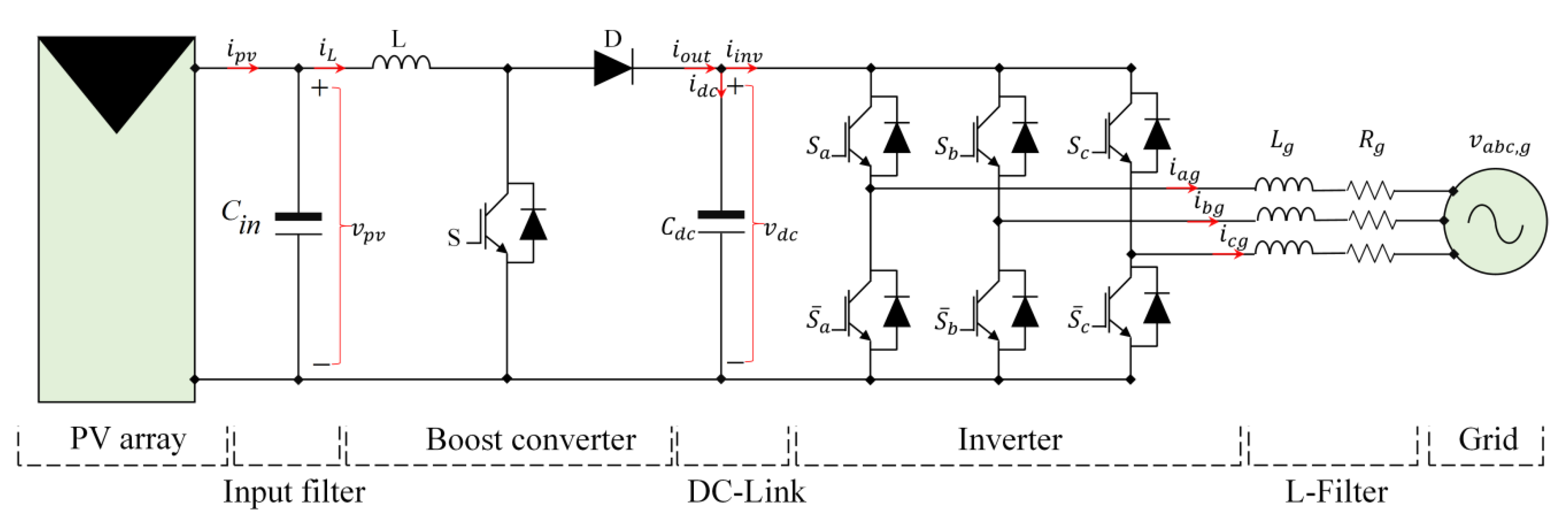Figure 2. PV array characteristics for different solar irradiation values: (a) I–V; (b) P–V.
Figure 2. PV array characteristics for different solar irradiation values: (a) I–V; (b) P–V.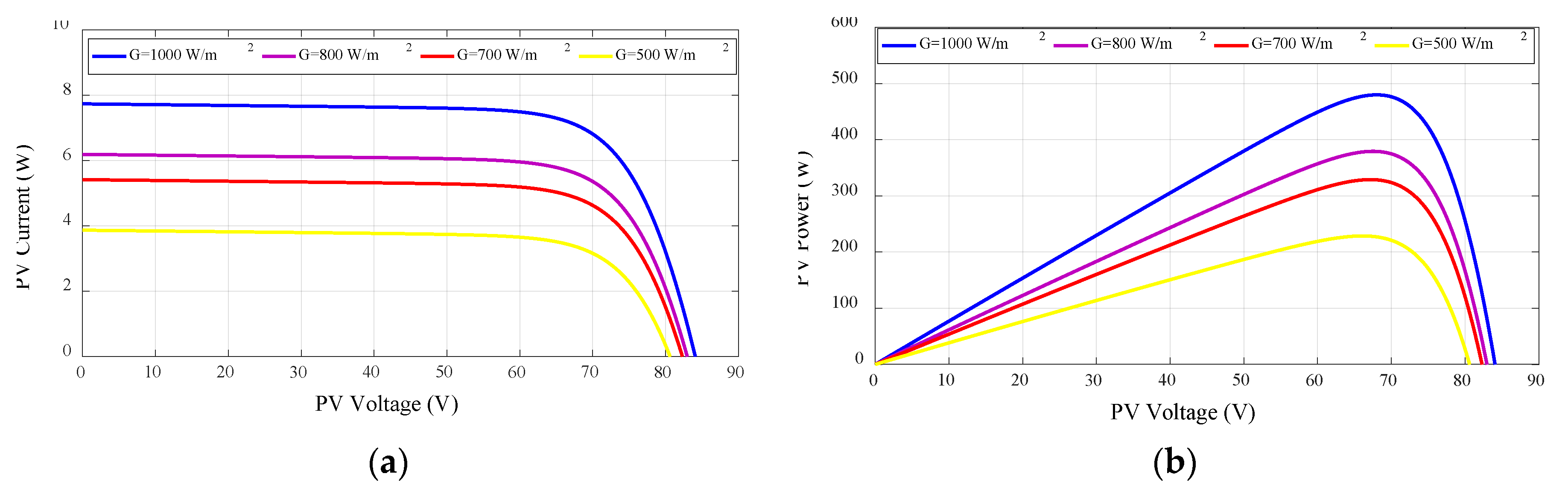Figure 3. PV array characteristics for different temperature values: (a) I–V; (b) P–V.
Figure 3. PV array characteristics for different temperature values: (a) I–V; (b) P–V.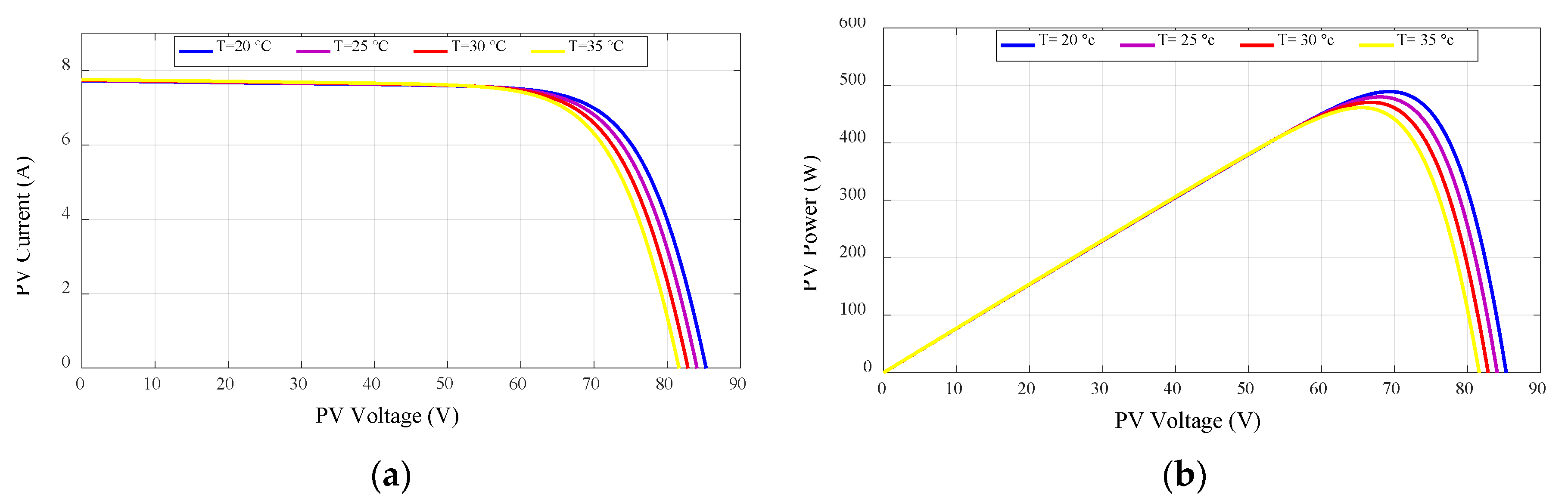Figure 4. Equivalent boost circuit: (a) on state; (b) off state.
Figure 4. Equivalent boost circuit: (a) on state; (b) off state.Figure 5. Approximation of sign function.
Figure 5. Approximation of sign function.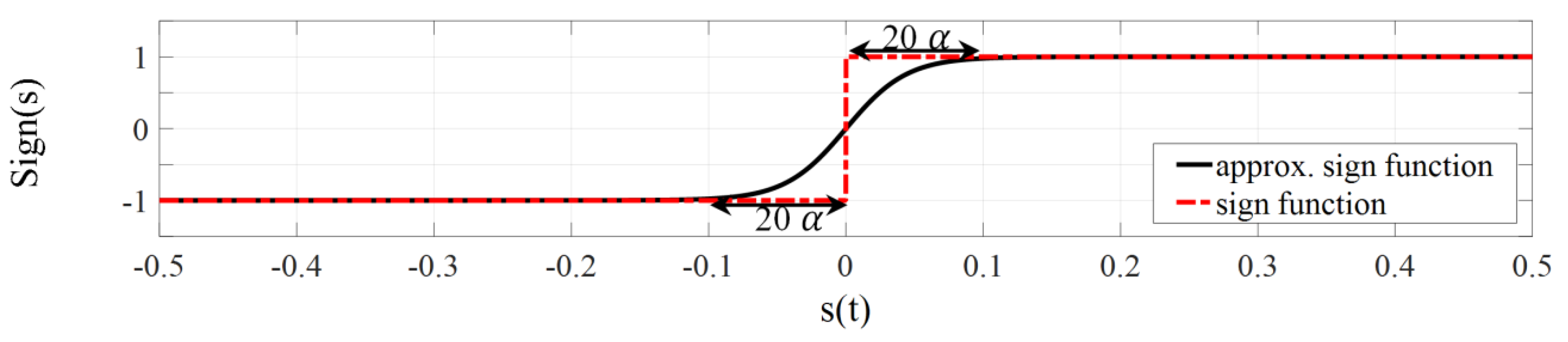Figure 6. Block diagram of proposed VO-MPPT.
Figure 6. Block diagram of proposed VO-MPPT.Figure 7. P&O-based V-MPPT flowchart.
Figure 7. P&O-based V-MPPT flowchart.Figure 8. Block diagram of the proposed DC-Link voltage controller based on ISMC theory.
Figure 8. Block diagram of the proposed DC-Link voltage controller based on ISMC theory.Figure 9. The proposed VOC based on ISMC.
Figure 9. The proposed VOC based on ISMC.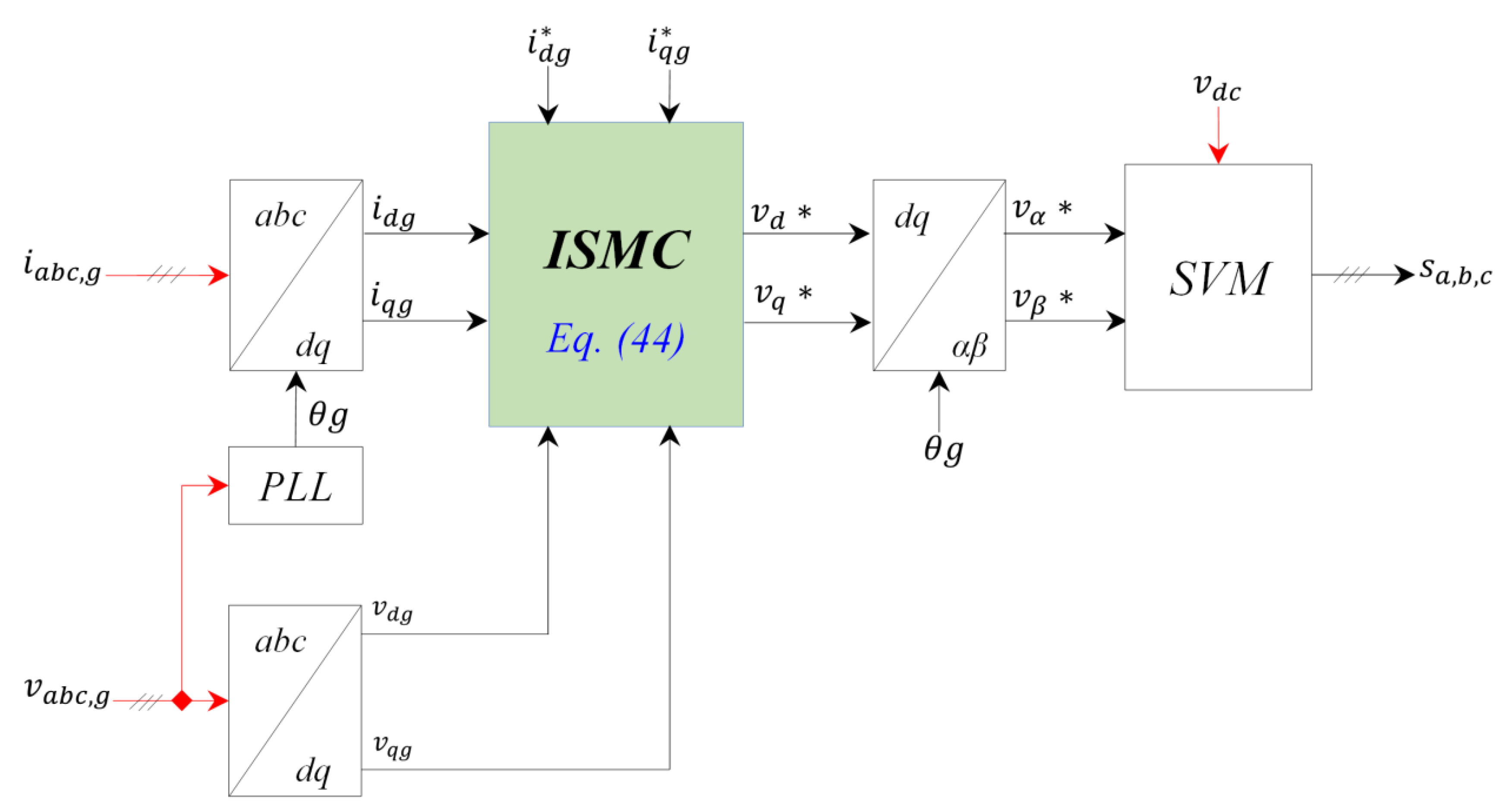Figure 11. Simulation results of grid-tied PV system with the conventional control scheme; under irradiation changes. (a) PV Voltage; (b) PV Current; (c) PV Power; (d) DC−Link Voltage; (e) Id Current and (f) Iq Current.
Figure 11. Simulation results of grid-tied PV system with the conventional control scheme; under irradiation changes. (a) PV Voltage; (b) PV Current; (c) PV Power; (d) DC−Link Voltage; (e) Id Current and (f) Iq Current.Figure 12. Simulation results of grid-tied PV system with the proposed control scheme; under irradiation changes. (a) PV Voltage; (b) PV Current; (c) PV Power; (d) DC−Link Voltage; (e) Id Current and (f) Iq Current.
Figure 12. Simulation results of grid-tied PV system with the proposed control scheme; under irradiation changes. (a) PV Voltage; (b) PV Current; (c) PV Power; (d) DC−Link Voltage; (e) Id Current and (f) Iq Current.Figure 13. PV voltage with conventional and proposed VO-MPPT methods.
Figure 13. PV voltage with conventional and proposed VO-MPPT methods.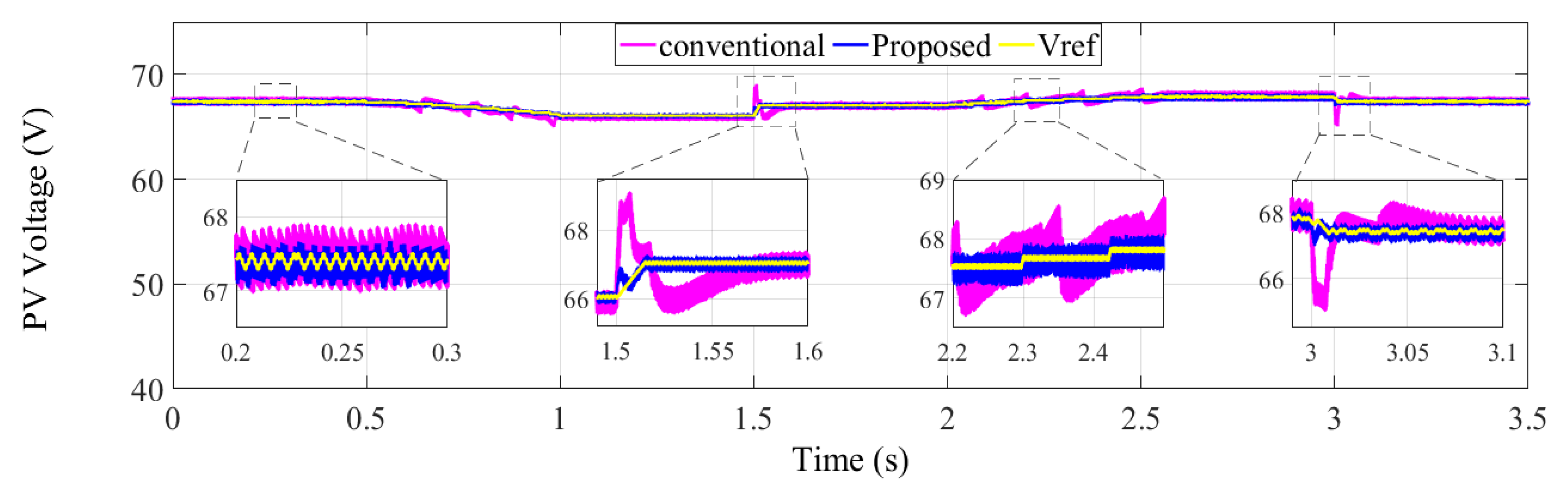Figure 14. Extracted PV power with conventional and proposed VO-MPPT methods.
Figure 14. Extracted PV power with conventional and proposed VO-MPPT methods.Figure 15. DC-Link voltage response with conventional and proposed methods.
Figure 15. DC-Link voltage response with conventional and proposed methods.Figure 16. Grid currents and their zoom waveforms: (a) conventional control scheme; (b) proposed control schemes; under irradiation changes.
Figure 16. Grid currents and their zoom waveforms: (a) conventional control scheme; (b) proposed control schemes; under irradiation changes.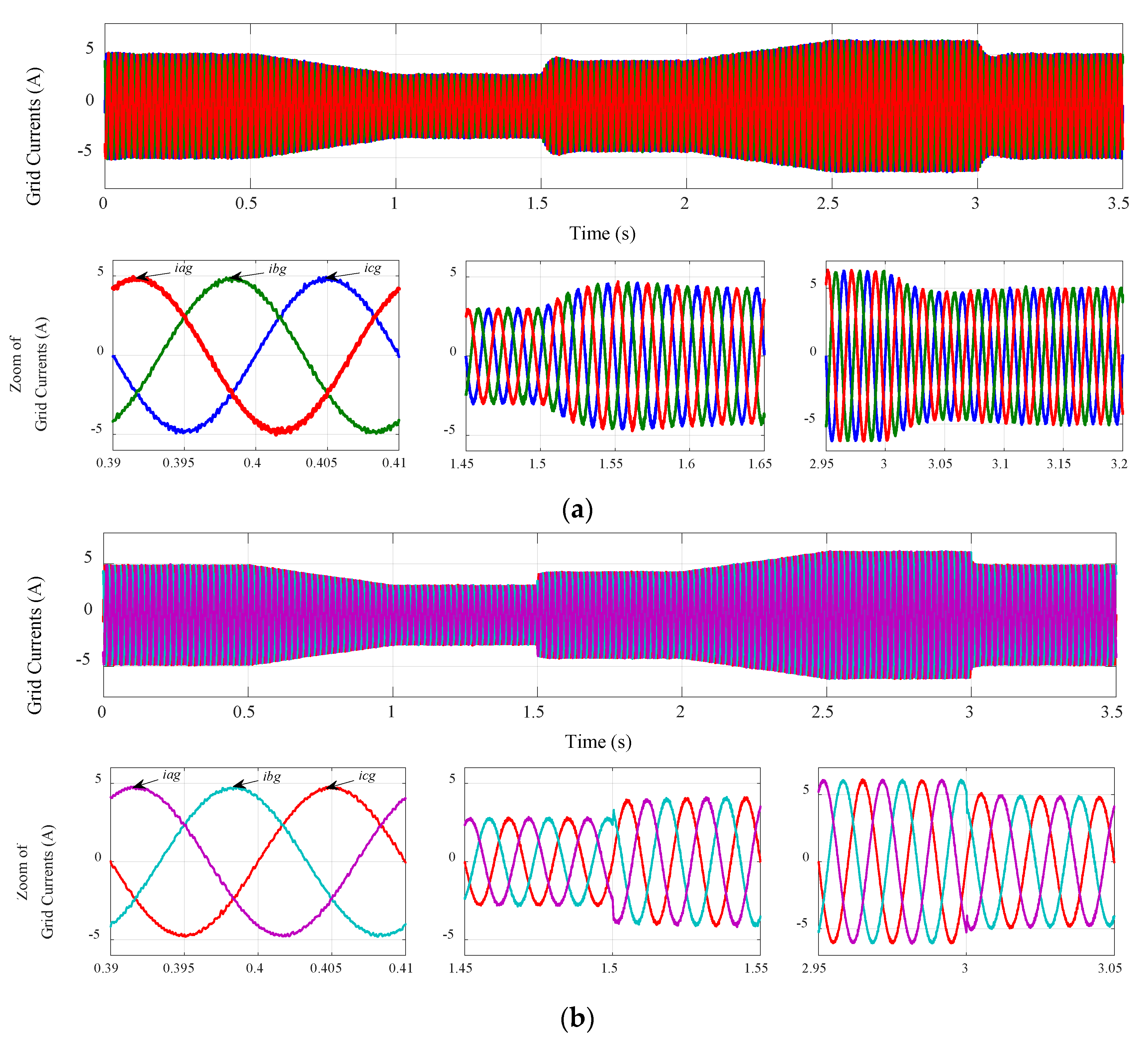Figure 17. Comparison of: (a) grid current THD%; (b) grid current ripples; under irradiation changes.
Figure 17. Comparison of: (a) grid current THD%; (b) grid current ripples; under irradiation changes.Table 1. Comparison results for MPPT methods.
Table 1. Comparison results for MPPT methods.
MPPT TechniqueStep Change in Irradiation 500→700 W/m²Step Change in Irradiation 1000→800 W/m²Linear Changes in Irradiation 800→500 W/m²
and 700→1000 W/m²
Power Oscillation (W)Response Time (s)Power Oscillation (W)Response Time (s)Tracking Accuracy
Conventional VO-MPPT0.170.0220.230.02low
Proposed VO-MPPTLess than 0.030.00850.0510.0061Very good
Table 2. Comparison results for DC-Link regulation.
Table 2. Comparison results for DC-Link regulation.